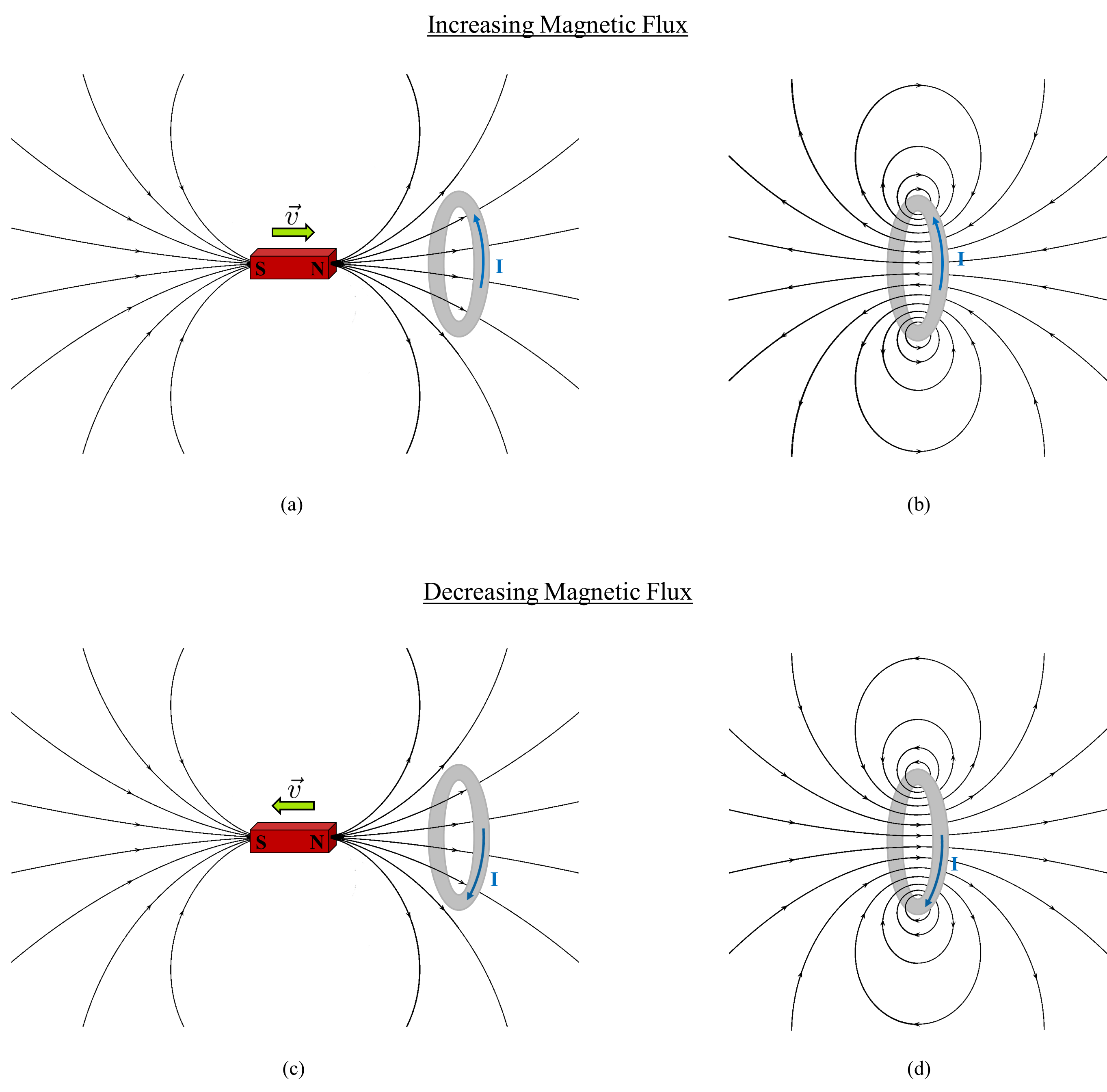# Lenz’s Law¶

Through a series of experiments in 1831 Michael Faraday came to the realization that changing magnetic fields create electric fields. Two years later, Heinrich Lenz formulated Lenz’s Law, which characterizes the direction of the currents induced in a conductor by these time varying magnetic fields.

A convenient way to quantify the strength of the magnetic field in a particular region is the magnetic flux ($$\Phi_{\mathbf{B}}$$),

(73)${\boldsymbol \Phi_b} = \int_A {\bf b} \cdot \hat {\bf{n}} \, da$

which provides a measure of the magnetic flux density over a given area.

(74)$\mathcal{E} = - \, \frac{\partial {\boldsymbol \Phi_b}}{\partial t}$

shows that any variation in the magnetic flux produces an electromotive force (emf, $$\mathcal{E}$$). This emf creates electrical currents within those bodies which are subjected to the time varying flux. The amplitude of the induced current is dependent on the strength of the emf and the conductivity of the material, while the direction of the induced current is characterized by Lenz’s Law.

Lenz’s Law states that the induced current will flow in such a direction that its secondary or induced magnetic fields act to oppose the observed change in magnetic flux. Simply put, “nature abhors a change in flux” so the induced current flows in such a manner to cancel out the change [Gri99]. This is the reason for the negative sign in Faraday’s Law, equation (74). Fig. 38 and the demonstration linked below provide visual illustrations of Lenz’s Law.Fig. 38 In panel (a) we see a situation in which the magnetic flux through the loop is increasing as a function of time. The induced current direction and the secondary magnetic field which opposes the increase in flux are shown in panel (b). Similarly, panel (c) shows when the magnetic flux through the loop is decreasing as a function of time and panel (d) demonstrates the direction of the induced current and secondary magnetic field. (Figure was created by M. Mitchell using the following Wikimedia Commons images: VFPt_dipole and VFPt ringcurrentNoLoop both of which are licensed under Creative Commons Attribution-Share Alike 3.0 Unported.)

Illustrative Demo:

Thanks to the Technical Services Group (TSG) at MIT’s Department of Physics for this great demo!